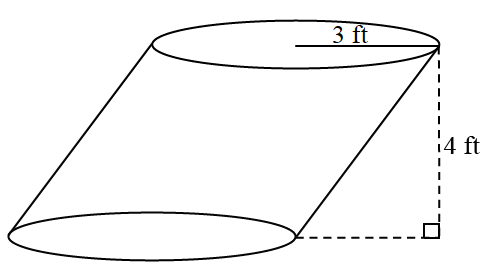### Home > CCG > Chapter 9 > Lesson 9.2.2 > Problem9-83

9-83.Compute the volume of the figure at right.

This is an oblique cylinder. So visualize this cylinder as circular pieces of paper stacked one on each other.
Could these pages be slid together to form a regular cylinder (height is $90°$ to the base)?
Would the area of the base stay the same? Would the height stay the same?

Calculate the new cylinder's volume using the known height and radius and the equation $V=\pi r^2h$.

$\text{Exact are of a cylinder}=36\pi\text{ ft}^3$
$\text{Approximate area}\approx113.10\text{ ft}^3$